moveit2 The MoveIt Motion Planning Framework for ROS 2.
unittest_trajectory_blender_transition_window.cpp File Reference
`#include <memory>`
`#include <gtest/gtest.h>`
`#include <moveit/kinematic_constraints/utils.h>`
`#include <moveit/robot_model/robot_model.h>`
`#include <moveit/robot_model_loader/robot_model_loader.h>`
`#include <moveit/robot_state/conversions.h>`
`#include <tf2_eigen/tf2_eigen.hpp>`
`#include <tf2_geometry_msgs/tf2_geometry_msgs.hpp>`
`#include <pilz_industrial_motion_planner_testutils/command_types_typedef.h>`
`#include <pilz_industrial_motion_planner_testutils/sequence.h>`
`#include <pilz_industrial_motion_planner_testutils/xml_testdata_loader.h>`
`#include <pilz_industrial_motion_planner/joint_limits_aggregator.h>`
`#include <pilz_industrial_motion_planner/trajectory_blend_request.h>`
`#include <pilz_industrial_motion_planner/trajectory_blend_response.h>`
`#include <pilz_industrial_motion_planner/trajectory_blender_transition_window.h>`
`#include <pilz_industrial_motion_planner/trajectory_generator_lin.h>`
`#include "test_utils.h"`
`#include <rclcpp/rclcpp.hpp>`
Include dependency graph for unittest_trajectory_blender_transition_window.cpp: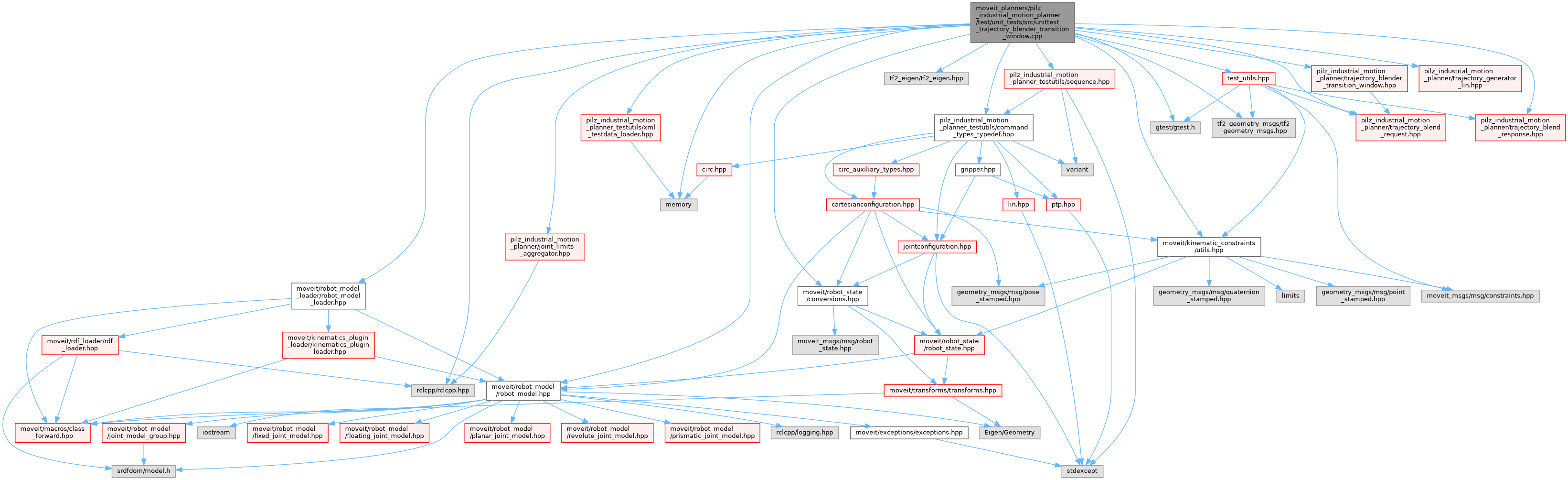Go to the source code of this file.

## Classes

class  TrajectoryBlenderTransitionWindowTest

## Functions

TEST_F (TrajectoryBlenderTransitionWindowTest, testInvalidGroupName)
Tests the blending of two trajectories with an invalid group name. More...

Tests the blending of two trajectories with an invalid target link. More...

Tests the blending of two trajectories with a negative blending radius. More...

Tests the blending of two trajectories with zero blending radius. More...

TEST_F (TrajectoryBlenderTransitionWindowTest, testDifferentSamplingTimes)
Tests the blending of two trajectories with differenent sampling times. More...

TEST_F (TrajectoryBlenderTransitionWindowTest, testNonUniformSamplingTime)
Tests the blending of two trajectories with one trajectory having non-uniform sampling time (apart from the last sample, which is ignored). More...

TEST_F (TrajectoryBlenderTransitionWindowTest, testNotIntersectingTrajectories)
Tests the blending of two trajectories which do not intersect. More...

TEST_F (TrajectoryBlenderTransitionWindowTest, testNonStationaryPoint)
Tests the blending of two cartesian trajectories with the shared point (last point of first, first point of second trajectory) having a non-zero velocity. More...

Tests the blending of two cartesian trajectories where the first trajectory is completely within the sphere defined by the blend radius. More...

Tests the blending of two cartesian trajectories where the second trajectory is completely within the sphere defined by the blend radius. More...

TEST_F (TrajectoryBlenderTransitionWindowTest, testLinLinBlending)
Tests the blending of two cartesian linear trajectories using robot model. More...

TEST_F (TrajectoryBlenderTransitionWindowTest, testOverlappingBlendTrajectories)
Tests the blending of two cartesian linear trajectories which have an overlap in the blending sphere using robot model. To be precise, the trajectories exactly lie on top of each other. More...

TEST_F (TrajectoryBlenderTransitionWindowTest, testNonLinearBlending)
Tests the blending of two cartesian trajectories which differ from a straight line. More...

int main (int argc, char **argv)

## ◆ main()

 int main ( int argc, char ** argv )

## ◆ TEST_F() [1/13]

 TEST_F ( TrajectoryBlenderTransitionWindowTest , testDifferentSamplingTimes )

Tests the blending of two trajectories with differenent sampling times.

Test Sequence:

1. Generate two linear trajectories with different sampling times.
2. Try to generate blending trajectory.

Expected Results:

1. Two linear trajectories generated.
2. Blending trajectory cannot be generated.

Definition at line 309 of file unittest_trajectory_blender_transition_window.cpp.

Here is the call graph for this function: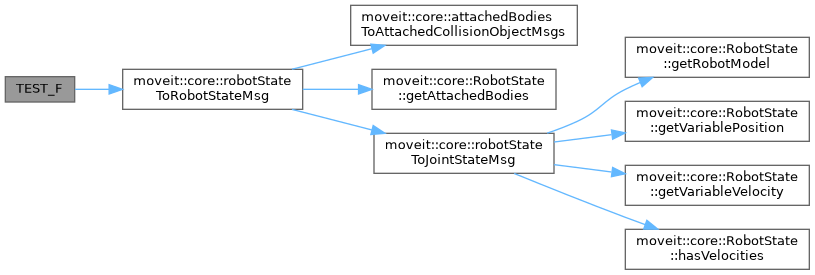## ◆ TEST_F() [2/13]

 TEST_F ( TrajectoryBlenderTransitionWindowTest , testInvalidGroupName )

Tests the blending of two trajectories with an invalid group name.

Test Sequence:

1. Generate two linear trajectories.
2. Try to generate blending trajectory with invalid group name.

Expected Results:

1. Two linear trajectories generated.
2. Blending trajectory cannot be generated.

Definition at line 191 of file unittest_trajectory_blender_transition_window.cpp.

## ◆ TEST_F() [3/13]

 TEST_F ( TrajectoryBlenderTransitionWindowTest , testInvalidTargetLink )

Tests the blending of two trajectories with an invalid target link.

Test Sequence:

1. Generate two linear trajectories.
2. Try to generate blending trajectory with invalid target link.

Expected Results:

1. Two linear trajectories generated.
2. Blending trajectory cannot be generated.

Definition at line 220 of file unittest_trajectory_blender_transition_window.cpp.

## ◆ TEST_F() [4/13]

 TEST_F ( TrajectoryBlenderTransitionWindowTest , testLinLinBlending )

Tests the blending of two cartesian linear trajectories using robot model.

Test Sequence:

1. Generate two linear trajectories from the test data set.
2. Generate blending trajectory.
3. Check blending trajectory:
• for position, velocity, and acceleration bounds,
• for continuity in joint space,
• for continuity in cartesian space.

Expected Results:

1. Two linear trajectories generated.
2. Blending trajectory generated.
3. No bound is violated, the trajectories are continuous in joint and cartesian space.

Definition at line 538 of file unittest_trajectory_blender_transition_window.cpp.

Here is the call graph for this function: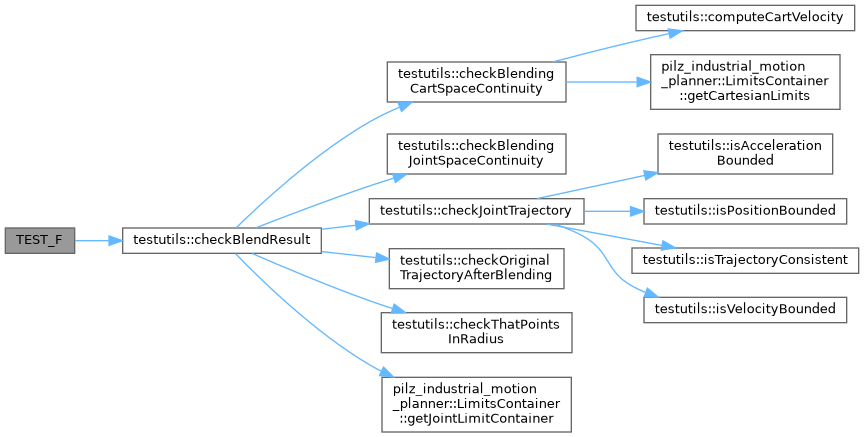## ◆ TEST_F() [5/13]

 TEST_F ( TrajectoryBlenderTransitionWindowTest , testNegativeRadius )

Tests the blending of two trajectories with a negative blending radius.

Test Sequence:

1. Generate two linear trajectories.
2. Try to generate blending trajectory with negative blending radius.

Expected Results:

1. Two linear trajectories generated.
2. Blending trajectory cannot be generated.

Definition at line 250 of file unittest_trajectory_blender_transition_window.cpp.

## ◆ TEST_F() [6/13]

 TEST_F ( TrajectoryBlenderTransitionWindowTest , testNonLinearBlending )

Tests the blending of two cartesian trajectories which differ from a straight line.

Test Sequence:

1. Generate two trajectories from the test data set.
2. Add scaled sine function to cartesian trajectories, such that start and end state remain unchanged; generate resulting joint trajectories using a time scaling in order to preserve joint velocity limits.
3. Generate blending trajectory.
4. Check blending trajectory:
• for position, velocity, and acceleration bounds,
• for continuity in joint space,
• for continuity in cartesian space.

Expected Results:

1. Two trajectories generated.
2. Modified joint trajectories generated.
3. Blending trajectory generated.
4. No bound is violated, the trajectories are continuous in joint and cartesian space.

Definition at line 627 of file unittest_trajectory_blender_transition_window.cpp.

Here is the call graph for this function: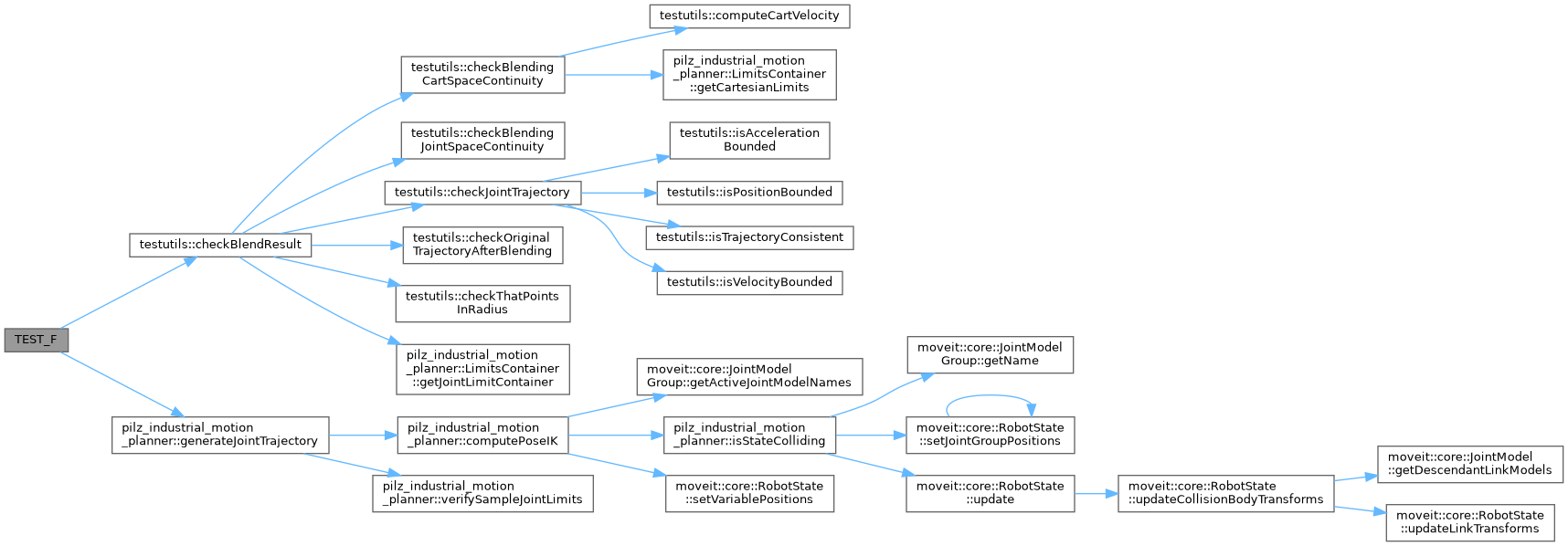## ◆ TEST_F() [7/13]

 TEST_F ( TrajectoryBlenderTransitionWindowTest , testNonStationaryPoint )

Tests the blending of two cartesian trajectories with the shared point (last point of first, first point of second trajectory) having a non-zero velocity.

Test Sequence:

1. Generate two trajectories from the test data set.
2. Generate blending trajectory modify the shared point to have velocity. Expected Results:
1. Two trajectories generated.
2. Blending trajectory cannot be generated.

Definition at line 427 of file unittest_trajectory_blender_transition_window.cpp.

## ◆ TEST_F() [8/13]

 TEST_F ( TrajectoryBlenderTransitionWindowTest , testNonUniformSamplingTime )

Tests the blending of two trajectories with one trajectory having non-uniform sampling time (apart from the last sample, which is ignored).

Test Sequence:

1. Generate two linear trajectories and corrupt uniformity of sampling time.
2. Try to generate blending trajectory.

Expected Results:

1. Two linear trajectories generated.
2. Blending trajectory cannot be generated.

Definition at line 362 of file unittest_trajectory_blender_transition_window.cpp.

## ◆ TEST_F() [9/13]

 TEST_F ( TrajectoryBlenderTransitionWindowTest , testNotIntersectingTrajectories )

Tests the blending of two trajectories which do not intersect.

Test Sequence:

1. Generate two trajectories from valid test data set.
2. Replace the second trajectory by the first one.
1. Try to generate blending trajectory.

Expected Results:

1. Two trajectories generated.
2. Two trajectories that do not intersect.
1. Blending trajectory cannot be generated.

Definition at line 396 of file unittest_trajectory_blender_transition_window.cpp.

## ◆ TEST_F() [10/13]

 TEST_F ( TrajectoryBlenderTransitionWindowTest , testOverlappingBlendTrajectories )

Tests the blending of two cartesian linear trajectories which have an overlap in the blending sphere using robot model. To be precise, the trajectories exactly lie on top of each other.

Test Sequence:

1. Generate two linear trajectories from the test data set. Set goal of second traj to start of first traj.
2. Generate blending trajectory.
3. Check blending trajectory:
• for position, velocity, and acceleration bounds,
• for continuity in joint space,
• for continuity in cartesian space.

Expected Results:

1. Two linear trajectories generated.
2. Blending trajectory generated.
3. No bound is violated, the trajectories are continuous in joint and cartesian space.

Definition at line 581 of file unittest_trajectory_blender_transition_window.cpp.

Here is the call graph for this function: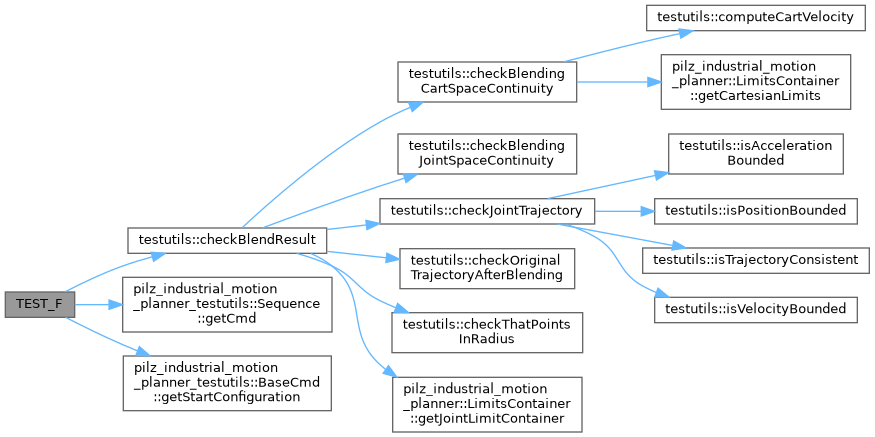## ◆ TEST_F() [11/13]

 TEST_F ( TrajectoryBlenderTransitionWindowTest , testTraj1InsideBlendRadius )

Tests the blending of two cartesian trajectories where the first trajectory is completely within the sphere defined by the blend radius.

Test Sequence:

1. Generate two trajectories from the test data set.
2. Generate blending trajectory with a blend_radius larger than the smaller trajectory.

Expected Results:

1. Two trajectories generated.
2. Blending trajectory cannot be generated.

Definition at line 464 of file unittest_trajectory_blender_transition_window.cpp.

## ◆ TEST_F() [12/13]

 TEST_F ( TrajectoryBlenderTransitionWindowTest , testTraj2InsideBlendRadius )

Tests the blending of two cartesian trajectories where the second trajectory is completely within the sphere defined by the blend radius.

Test Sequence:

1. Generate two trajectories from the test data set.
2. Generate blending trajectory with a blend_radius larger than the smaller trajectory.

Expected Results:

1. Two trajectories generated.
2. Blending trajectory cannot be generated.

Definition at line 501 of file unittest_trajectory_blender_transition_window.cpp.

## ◆ TEST_F() [13/13]

 TEST_F ( TrajectoryBlenderTransitionWindowTest , testZeroRadius )

Tests the blending of two trajectories with zero blending radius.

Test Sequence:

1. Generate two linear trajectories.
2. Try to generate blending trajectory with zero blending radius.

Expected Results:

1. Two linear trajectories generated.
2. Blending trajectory cannot be generated.

Definition at line 279 of file unittest_trajectory_blender_transition_window.cpp.